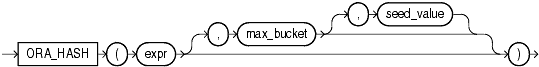## ORA_HASH

Syntax

ora_hash::=Description of the illustration ora_hash.gif

Purpose

`ORA_HASH` is a function that computes a hash value for a given expression. This function is useful for operations such as analyzing a subset of data and generating a random sample.

• The `expr` argument determines the data for which you want Oracle Database to compute a hash value. There are no restrictions on the type or length of data represented by `expr`, which commonly resolves to a column name.

• The optional `max_bucket` argument determines the maximum bucket value returned by the hash function. You can specify any value between 0 and 4294967295. The default is 4294967295.

• The optional `seed_value` argument enables Oracle to produce many different results for the same set of data. Oracle applies the hash function to the combination of `expr` and `seed_value`. You can specify any value between 0 and 4294967295. The default is 0.

The function returns a `NUMBER` value.

Examples

The following example creates a hash value for each combination of customer ID and product ID in the `sh.sales` table, divides the hash values into a maximum of 100 buckets, and returns the sum of the `amount_sold` values in the first bucket (bucket 0). The third argument (5) provides a seed value for the hash function. You can obtain different hash results for the same query by changing the seed value.

```SELECT SUM(amount_sold) FROM sales
WHERE ORA_HASH(CONCAT(cust_id, prod_id), 99, 5) = 0;

SUM(AMOUNT_SOLD)
----------------
7315

```

The following example retrieves a subset of the data in the `sh.sales` table by specifying 10 buckets (0 to 9) and then returning the data from bucket 1. The expected subset is about 10% of the rows (the `sales` table has 960 rows):

```SELECT * FROM sales
WHERE ORA_HASH(cust_id, 9) = 1;

PROD_ID    CUST_ID TIME_ID   C   PROMO_ID QUANTITY_SOLD AMOUNT_SOLD
---------- ---------- --------- - ---------- ------------- -----------
2510       6950 01-FEB-98 S       9999             2          78
9845       9700 04-FEB-98 C       9999            17         561
3445      33530 07-FEB-98 T       9999             2         170
. . .
740      22200 13-NOV-00 S       9999             4         156
9425       4750 29-NOV-00 I       9999            11         979
1675      46750 29-NOV-00 S       9999            19        1121
97 rows selected.
```